Скачать презентацию Section 6 6 Limiting Reactants What happens if you

1b8a61d49c1269f0c3903b5d53cf23f7.ppt

• Количество слайдов: 8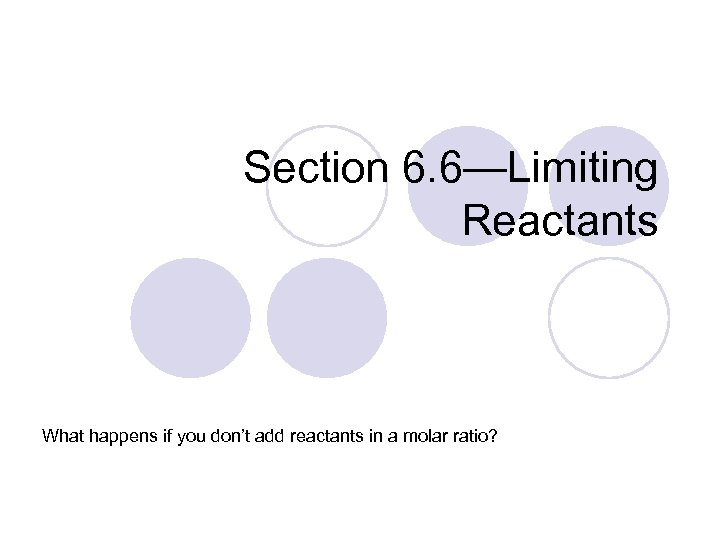Section 6. 6—Limiting Reactants What happens if you don’t add reactants in a molar ratio?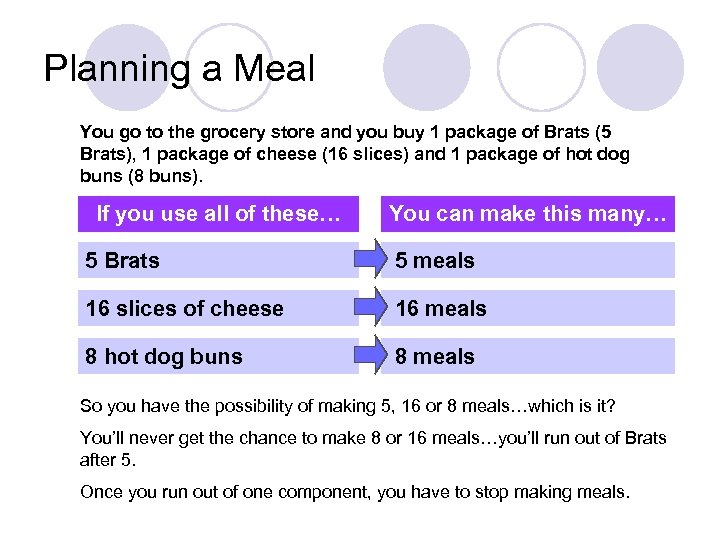Planning a Meal You go to the grocery store and you buy 1 package of Brats (5 Brats), 1 package of cheese (16 slices) and 1 package of hot dog buns (8 buns). If you use all of these… You can make this many… 5 Brats 5 meals 16 slices of cheese 16 meals 8 hot dog buns 8 meals So you have the possibility of making 5, 16 or 8 meals…which is it? You’ll never get the chance to make 8 or 16 meals…you’ll run out of Brats after 5. Once you run out of one component, you have to stop making meals.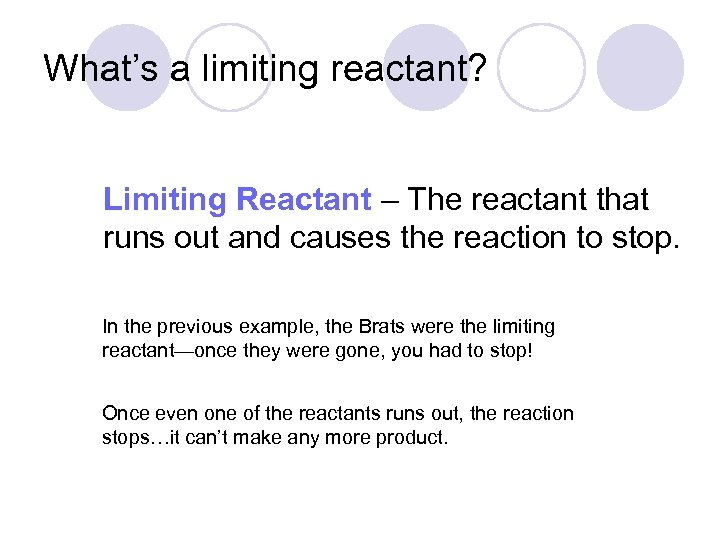What’s a limiting reactant? Limiting Reactant – The reactant that runs out and causes the reaction to stop. In the previous example, the Brats were the limiting reactant—once they were gone, you had to stop! Once even one of the reactants runs out, the reaction stops…it can’t make any more product.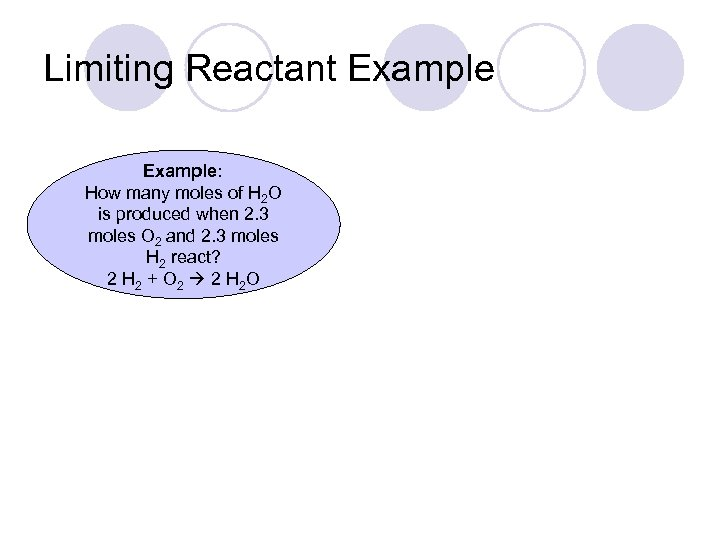Limiting Reactant Example: How many moles of H 2 O is produced when 2. 3 moles O 2 and 2. 3 moles H 2 react? 2 H 2 + O 2 2 H 2 O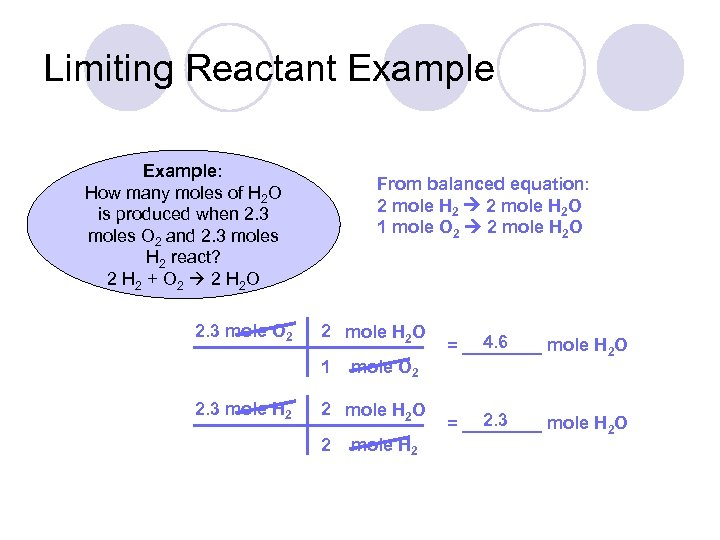Limiting Reactant Example: How many moles of H 2 O is produced when 2. 3 moles O 2 and 2. 3 moles H 2 react? 2 H 2 + O 2 2 H 2 O 2. 3 mole O 2 From balanced equation: 2 mole H 2 O 1 mole O 2 2 mole H 2 O 1 2. 3 mole H 2 mole O 2 2 mole H 2 O 2 mole H 2 4. 6 = ____ mole H 2 O 2. 3 = ____ mole H 2 O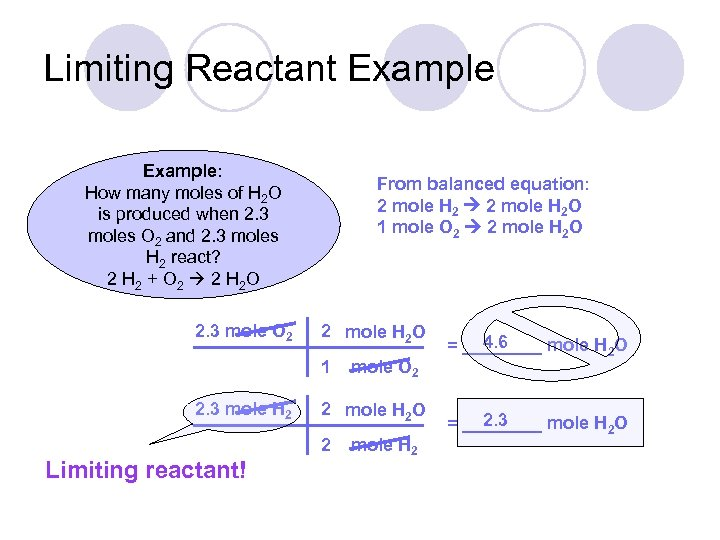Limiting Reactant Example: How many moles of H 2 O is produced when 2. 3 moles O 2 and 2. 3 moles H 2 react? 2 H 2 + O 2 2 H 2 O 2. 3 mole O 2 From balanced equation: 2 mole H 2 O 1 mole O 2 2 mole H 2 O 1 2. 3 mole H 2 2 mole H 2 O 2 Limiting reactant! mole O 2 mole H 2 4. 6 = ____ mole H 2 O 2. 3 = ____ mole H 2 O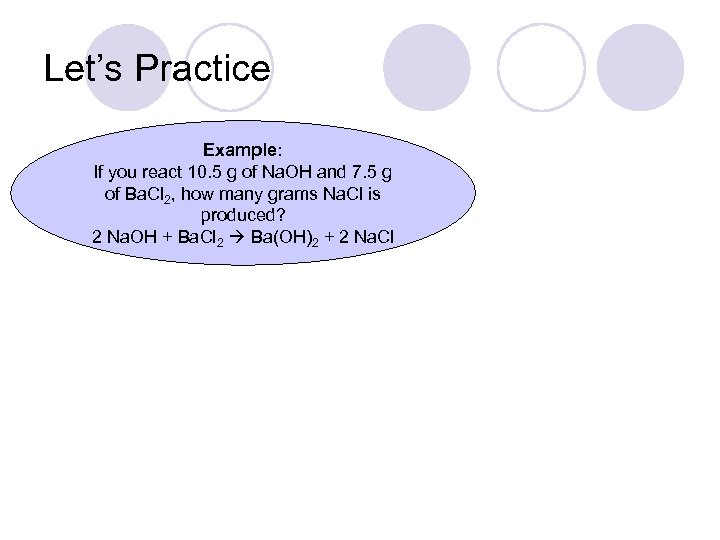Let’s Practice Example: If you react 10. 5 g of Na. OH and 7. 5 g of Ba. Cl 2, how many grams Na. Cl is produced? 2 Na. OH + Ba. Cl 2 Ba(OH)2 + 2 Na. Cl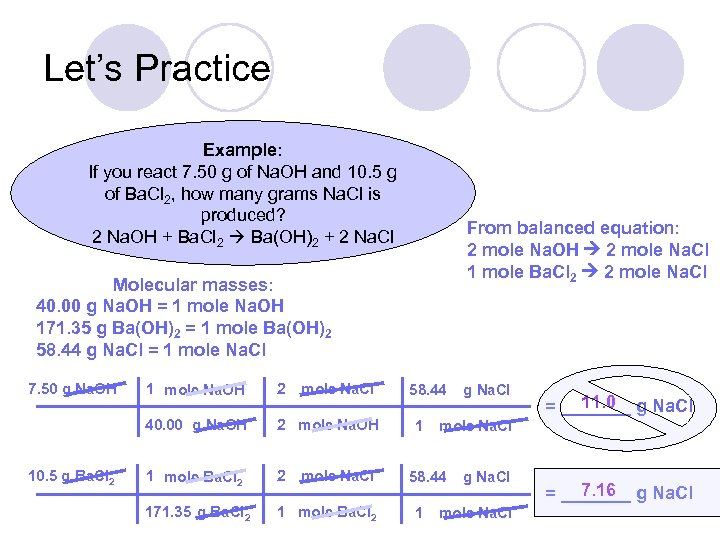Let’s Practice Example: If you react 7. 50 g of Na. OH and 10. 5 g of Ba. Cl 2, how many grams Na. Cl is produced? 2 Na. OH + Ba. Cl 2 Ba(OH)2 + 2 Na. Cl From balanced equation: 2 mole Na. OH 2 mole Na. Cl 1 mole Ba. Cl 2 2 mole Na. Cl Molecular masses: 40. 00 g Na. OH = 1 mole Na. OH 171. 35 g Ba(OH)2 = 1 mole Ba(OH)2 58. 44 g Na. Cl = 1 mole Na. Cl 7. 50 g Na. OH 2 40. 00 g Na. OH 10. 5 g Ba. Cl 2 1 mole Na. OH mole Na. Cl 2 mole Na. OH 1 mole Ba. Cl 2 2 171. 35 g Ba. Cl 2 1 mole Ba. Cl 2 mole Na. Cl 58. 44 1 11. 0 = _______ g Na. Cl mole Na. Cl 58. 44 1 g Na. Cl mole Na. Cl 7. 16 = _______ g Na. Cl